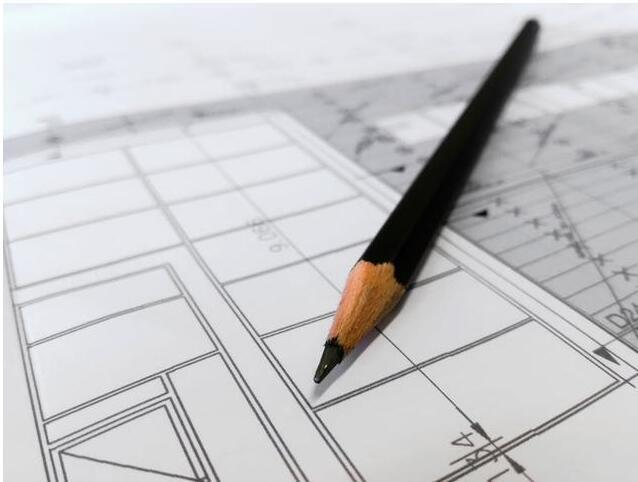# 大神总结：100个CAD快捷键+20个CAD制图技巧，学会你就逆天了！

1. 圆 <--> C

2. 点 <--> PO

3. 直线 <--> L

4. 圆弧 <--> A

5. 椭圆 <--> EL

6. 表格 <--> TB

7. 矩形 <--> REC

8. 面域 <--> REG

9. 创建块 <--> B

10. 插入块 <--> I

11. 多段线 <--> PL

12. 构造线 <--> XL

13. 图案填充 <--> H

14. 样条曲线 <--> SPL

15. 正多边形 <--> POL16. 线性标注 <--> DLI

17. 对齐标注 <--> DAL

18. 弧长标注 <--> DAR

19. 坐标标注 <--> DOR

20. 半径标注 <--> DRA

21. 折弯标注 <--> DJO

22. 直径标注 <--> DDI

23. 角度标注 <--> DAN

24. 快速标注 <--> QDIM

25. 基线标注 <--> DBA

26. 连续标注 <--> DCO

27. 形位公差 <--> TOL

28. 标记圆心 <--> DCE

29. 折弯线性 <--> DJL

30. 编辑标注 <--> DED

31. 标注样式 <--> DST32. 删除 <--> E

33. 复制 <--> CO

34. 镜像 <--> MI

35. 偏移 <--> O

36. 阵列 <--> AR

37. 移动 <--> M

38. 旋转 <--> RO

39. 缩放 <--> SC

40. 拉伸 <--> S

41. 裁剪 <--> TR

42. 延伸 <--> EX

43. 打断 <--> BR

44. 合并 <--> J

45. 倒角 <--> CHA

46. 圆角 <--> F

47. 分解 <--> X

48. 多行文字 <--> MT

49. 单行文字 <--> DT

50. 修改文字 <--> ED

51. 查找替换 <--> FIND

52. 拼写检查 <--> SP53. 文字样式 <--> ST

54. 表格样式 <--> TS

55. 引线样式 <--> MLS

56. 图层管理 <--> LA

57. 图层状态 <--> LAS

58. 冻结图层 <--> layfrz

59. 关闭图层 <--> layoff

60. 锁定图层 <--> laylck

61. 解锁图层 <--> layulk

62.直线 <--> L

63.圆 <--> C

64.矩形 <--> REC

65.便移 <--> O

66.移动 <--> M

67.复制 <--> CO

68.旋转 <--> R

69.文字 <--> T

70.减切 <--> TR

80.多线段 <--> PL

81.删除 <--> E

82.匹配 <--> MA

83.返回 <--> U

84.图纸移动 <--> P

85.缩放 <--> Z

86.属性 <--> MO

87.面积统计 <--> LI

88.拉升 <--> S

89.比例缩放 <--> SC

90.扎开 <--> XCAD常用快捷键

91.颜色 <--> AIT+O+C（以下省AIT+O）

92.线型 <--> +N

93.图层 <--> +L

94.线宽 <--> +W

95.文字样式 <--> +S

96.表注样式 <--> +D

97.打印样式 <--> +Y

98.点样式 <--> +P

99.多线样式 <--> +M

100.单行文字 <--> OT

1.CTrl+N无效时之解决办法

2.Ctrl键无效之解决办法

3填充无效时之解决办法

4.加选无效时之解决办法

5.如果CAD里的系统变量被人无意更改或一参 数被人有意调整了怎么办？

6.鼠标中键不好用怎么办呀？

op--选项--显示--显示布局和模型选项卡

7.CAD技巧

OP选项--用户系统配置--绘图区域中使用捷8.图形里的圆不圆了咋办呀？

9.图形窗口中显示滚动条

op--显示--图形窗口中显示滚动条即可

10.保存的格式

OP--打开和保存--另存为2000格式

11.如果想下次打印的线型和这次的一样怎么办？

op选项--打印--添加打印列表

12.如果在标题栏显示路径不全怎么办？

op选项--打开和保存--在标题栏中显示完整路径（勾选 ）即可13.如何减少文件大小？

-purge，前面加个减号，清理的会更彻底些，

14.如何将自动保存的图形复原

AutoCAD将自动保存的图形存放到AUTO.SV$或AUTO?.SV$文件中，找到该文件将其改名为图形文件即可在AutoCAD中打开。

15.为什么不能显示汉字？或输入的汉字变成了问号

16.为什么输入的文字高度无法改变？

17.为什么有些图形能显示，却打印不出来？

18.DWG文件破坏了咋办呀？

19. 在布局中插入图框

20. 如何关闭CAD中的*BAK文件：